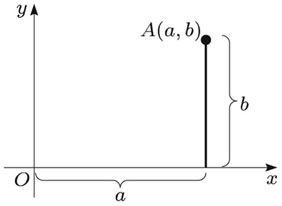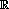Vectors and conics

This free course is available to start right now. Review the full course description and key learning outcomes and create an account and enrol if you want a free statement of participation.

Free course

1 Coordinate geometry: points, planes and lines

1.1 Points, lines and distances in two-dimensional Euclidean space

In coordinate geometry we generally use rectangular (or Cartesian) coordinate axes, as illustrated below, to describe the Euclidean plane. We can represent any point in the plane uniquely by an ordered pair of coordinates (a, b); that is, any point in the plane has precisely one possible pair of coordinates with respect to the chosen axes.This gives a one-one correspondence between the points of the plane and ordered pairs of real numbers. Often we do not bother to distinguish explicitly between the points and their representation as ordered pairs; we simply write (a, b) to denote the point A.

The plane together with an origin and a pair of x-, y-axes is often called two-dimensional Euclidean space, denoted by the symbol2.

M208_1